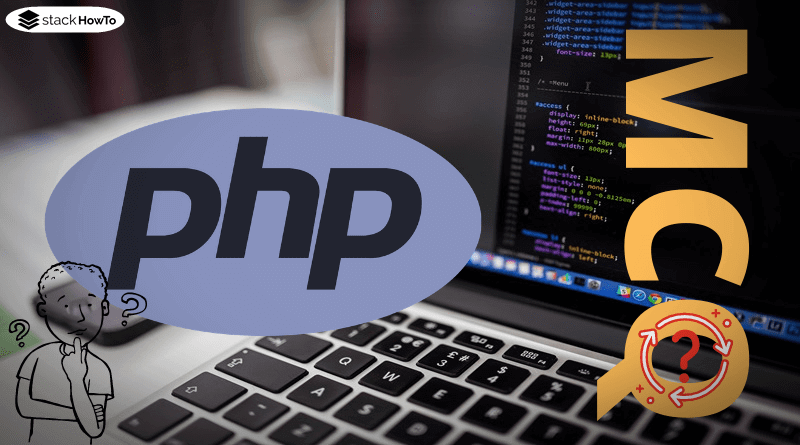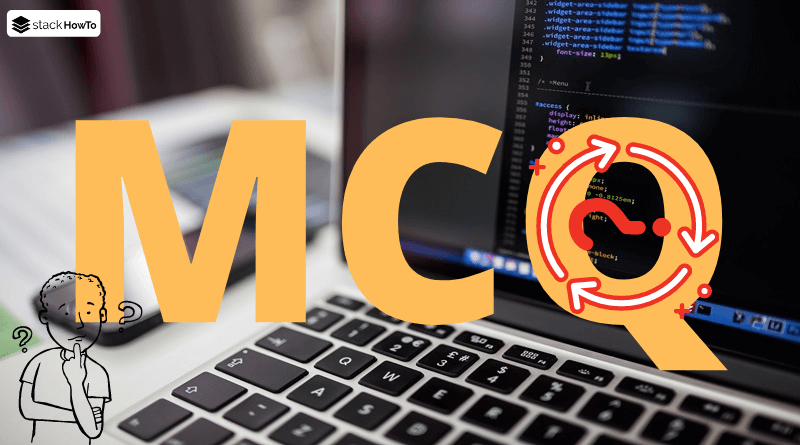php

# How to Convert Decimal to Binary in PHP?

In order to convert from the decimal value to the binary value, decbin() is used. The function expects an integer and returns the binary value as a string. The string of the binary number always starts with the first one, so there are no leading zeros.

###### Example :
```<?php
\$a = decbin(9);
\$b = decbin(15);
\$c = decbin(-15);
var_dump(\$a, \$b, \$c);
?>```

Output:

```string(4) "1001"
string(4) "1111"
string(64) "1111111111111111111111111111111111111111111111111111111111110001"```

The conversion can also be done with sprintf(). The string “%032b” must be transferred to the function as the first parameter and the decimal value as the second parameter. In the string “%032b” the number of digits of the resulting binary number is represented by “32” and can be changed as required. It is also possible to just pass “%b”. In this case, the number of digits is dynamic and the result is identical to decbin(). It is also possible to insert any additional text into the string, which then becomes part of the output (only a percentage sign should not be used as part of the text).

###### Example :
```<?php
\$a = sprintf('%b', 9); // Similar to decbin()
\$b = sprintf('%032b', 9); // at least 32 characters
\$c = sprintf('%064b', 9); // at least 64 characters
\$d = sprintf('The binary number is %b.', 9); // Binary number with text
\$e = sprintf('%02b', 1024); // at least 2 characters for a binary number with 11 characters
var_dump(\$a, \$b, \$c, \$d, \$e);
?>```

Output:

```string(4) "1001"
string(32) "00000000000000000000000000001001"
string(64) "0000000000000000000000000000000000000000000000000000000000001001"
string(26) "The binary number is 1001."
string(11) "10000000000"```PHP MCQ – Multiple Choice Questions and Answers – Basics – Part 1This collection of PHP Multiple Choice Questions and Answers (MCQs): Quizzes & Practice Tests with Answer focuses on “PHP basic”.   1. In PHP, variables…Read MoreMCQPractice competitive and technical Multiple Choice Questions and Answers (MCQs) with simple and logical explanations to prepare for tests and interviews.Read More